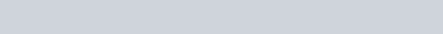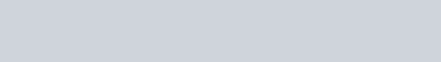# Sound classsification with YAMNet

In this tutorial, we will learn how to load and use the YAMNet model as an inference to classify cat and dogs sound.

## What is YAMNet?

The MobileNetV1 depthwise-separable convolution architecture is used by YAMNet, a pre-trained neural network. It can take an audio waveform as input and forecast each of the 521 audio events in the AudioSet corpus independently.

Internally, the model separates the audio signal into “frames” and processes batches of these frames. This version of the model extracts one frame every 0.48 seconds and utilizes frames that are 0.96 seconds long.

A 1-D float 32 Tensor or NumPy array containing a waveform of any length encoded as single-channel (mono) 16 kHz samples in the range [-1.0, +1.0], is accepted by the model. This lesson includes code that will assist you in converting WAV files to a supported format.

The model produces three outputs: class scores, embeddings (which will be used for transfer learning), and a log mel spectrogram. More information is available here.

The 1,024-dimensional embedding output of YAMNet may be used as a high-level feature extractor. The input characteristics of the base (YAMNet) model will be fed into your shallower model, which consists of one hidden tf.Keras. layers. Dense layer. The network will then be trained on a little quantity of data for audio classification without the need for a large amount of labeled data or end-to-end training.

### Import Libraries

Let’s import all the required libraries.

```import os
from IPython import display
import matplotlib.pyplot as plt
import numpy as np
import pandas as pd
import tensorflow as tf
import tensorflow_hub as hub
import tensorflow_io as tfio```

### Load YAMNet from TF Hub

To extract the embeddings from the sound files, you’ll utilize a pre-trained YAMNet from TF Hub.

It’s simple to load a model from TF Hub: choose the model, copy the URL, and use the load function.

```yamnet_model_ = 'https://tfhub.dev/google/yamnet/1'

Lets download Wav i.e sound file using tf.keras.util.get_file().

Code

```test_wav_file_name = tf.keras.utils.get_file('miaow_16k.wav',
cache_dir='./',
cache_subdir='test_data')

print(test_wav_file_name)```

Output### Load Audio Files

Let’s create a function that will load a WAV file, which then converts the WAV file into a float tensor and resample it 16kHz single-channel audio. Apply this function to test_wav_file_name which we created above. Then plot the soundwaves graph and play the audio file.

Code

```@tf.function
wav, sample_rate = tf.audio.decode_wav(
file,
desired_channels=1)
wav = tf.squeeze(wav, axis=-1)
sample_rate = tf.cast(sample_rate, dtype=tf.int64)
wav = tfio.audio.resample(wav, rate_in=sample_rate, rate_out=16000)
return wav

_ = plt.plot(testing_wav_data)

# Play the audio file.
display.Audio(testing_wav_data,rate=16000)```

Output### Load Class Mapping

Let’s load the class names that YAMNet can recognize. The mapping file is available in CSV format via yamnet model.class map path().

Code

```class_path = yamnet_model.class_map_path().numpy().decode('utf-8')

for name in class_names[:21]:
print(name)
print('...')```

Output### Run Inference

YAMNet delivers class scores at the frame level (i.e., 521 scores for every frame). The scores may be pooled per class over frames to produce clip-level predictions (e.g., using mean or max aggregation). This is done in the code below by scores np. mean(axis=0). Finally, to determine the highest-scoring class at the clip-level, take the sum of the 521 aggregated scores.

Code

```scores, embeddings, spectrogram = yamnet_model(testing_wav_data)
class_scores = tf.reduce_mean(scores, axis=0)
top_class = tf.argmax(class_scores)
infernce_class = class_names[top_class]

print(f'The main sound is: {infernce_class}')
print(f'The embeddings shape: {embeddings.shape}')```

Output

The model successfully predicted the sound of an animal. In this course, our aim is to improve the model’s accuracy for certain classes. Also, the model generated 13 embeddings, one for each frame.### ESC-50 Dataset

ESC-50 dataset, which is a tagged collection of 2,000 five-second long ambient audio recordings. The dataset is divided into 50 classes, each with 40 samples. Let’s Download the data and extract it.

Code

```_ = tf.keras.utils.get_file('esc-50.zip',
'https://github.com/karoldvl/ESC-50/archive/master.zip',
cache_dir='./',
cache_subdir='datasets',
extract=True)```

Output### Explore Dataset

Let’s explore the data using the panda’s library.

Code

```esc = './datasets/ESC-50-master/meta/esc50.csv'
base_data_path = './datasets/ESC-50-master/audio/'

Output### Filter Data

Let’s apply some filters to our dataset:-

• Select only dog and cat classes in the dataset.
• Assign dog mapping as 0 and cat as 1.

Code

```my_class = ['dog', 'cat']
mapcl= {'dog':0, 'cat':1}

filter_pd = pd_data[pd_data.category.isin(my_class)]

class_id = filter_pd['category'].apply(lambda name: mapcl[name])
filter_pd = filter_pd.assign(target=class_id)

full_path = filter_pd['filename'].apply(lambda row: os.path.join(base_data_path, row))
filter_pd = filter_pd.assign(filename=full_path)

Output### Load audio files and retrieve embedding

Let’s apply the load_wav_16k_mono and prepare the WAV data for the modeling.

When you extract embeddings from WAV data, you obtain an array with the shape (N, 1024), where N is the number of frames detected by YAMNet (one for every 0.48 seconds of audio).

Each frame will be used as one input in your model. As a result, you’ll need to make a new column with one frame per row. To properly represent these extra rows, you’ll also need to enlarge the labels and the fold column.

The original values are kept in the enlarged fold column. You can’t mix frames because if you do, you’ll wind up with bits of the same audio on separate splits, reducing the effectiveness of your validation and test procedures.

Code

```filenames = filter_pd['filename']
targets = filter_pd['target']
folds = filter_pd['fold']

main_ds = tf.data.Dataset.from_tensor_slices((filenames, targets, folds))
main_ds.element_spec```
```def load_wav_for_map(filename, label, fold):
return load_wav_16k_mono(filename), label, fold

main_ds.element_spec```

Output### Apply embeddings model

Let’s apply the embedding extraction model to wav data by creating the function ex_embedding().

Code

```def ex_embedding(wav_data, label, fold):
scores, embeddings, spectrogram = yamnet_model(wav_data)
num_embeddings = tf.shape(embeddings)
return (embeddings,
tf.repeat(label, num_embeddings),
tf.repeat(fold, num_embeddings))

main_ds = main_ds.map(ex_embedding).unbatch()
main_ds.element_spec```

Output### Split Data

Let’s split the data into train, valid, and test based on the column fold in the final dataset.

ESC-50 is divided into five cross-validation folds of uniform size, ensuring that clips from the same originating source are always in the same fold – see the ESC: Dataset for Environmental Sound Classification paper for additional information.

We will remove the fold column from the dataset since we are not going to use it during training.

Code

```cached_ds = main_ds.cache()
train = cached_ds.filter(lambda embedding, label, fold: fold < 4)
val = cached_ds.filter(lambda embedding, label, fold: fold == 4)
test = cached_ds.filter(lambda embedding, label, fold: fold == 5)

rm_fold_column = lambda embedding, label, fold: (embedding, label)

train = train.map(rm_fold_column)
val = val.map(rm_fold_column)
test = test.map(rm_fold_column)

train = train.cache().shuffle(1000).batch(32).prefetch(tf.data.AUTOTUNE)
val = val.cache().batch(32).prefetch(tf.data.AUTOTUNE)
test = test.cache().batch(32).prefetch(tf.data.AUTOTUNE)```

### Create Model

Let’s create a  Sequential model with one hidden layer and two outputs as cat and dog sounds.

Code

```model = tf.keras.Sequential([
tf.keras.layers.Input(shape=(1024), dtype=tf.float32,
name='input_embedding'),
tf.keras.layers.Dense(512, activation='relu'),
tf.keras.layers.Dense(len(my_classes))
], name='my_model')

model.summary()```

Output### Compile and Fit Model

Let’s compile and fit the Sequential model which we created above.

Code

```model.compile(loss=tf.keras.losses.SparseCategoricalCrossentropy(from_logits=True),
metrics=['accuracy'])

callback = tf.keras.callbacks.EarlyStopping(monitor='loss',
patience=3,
restore_best_weights=True)
history = model.fit(train_ds,
epochs=20,
validation_data=val,
callbacks=callback)```

Output

```Epoch 1/20
15/15 [==============================] - 6s 49ms/step - loss: 0.7811 - accuracy: 0.8229 - val_loss: 0.4866 - val_accuracy: 0.9125
Epoch 2/20
15/15 [==============================] - 0s 18ms/step - loss: 0.3385 - accuracy: 0.8938 - val_loss: 0.2185 - val_accuracy: 0.8813
Epoch 3/20
15/15 [==============================] - 0s 18ms/step - loss: 0.3091 - accuracy: 0.9021 - val_loss: 0.4290 - val_accuracy: 0.8813
Epoch 4/20
15/15 [==============================] - 0s 18ms/step - loss: 0.5354 - accuracy: 0.9062 - val_loss: 0.2074 - val_accuracy: 0.9125
Epoch 5/20
15/15 [==============================] - 0s 18ms/step - loss: 0.4651 - accuracy: 0.9333 - val_loss: 0.6857 - val_accuracy: 0.8813
Epoch 6/20
15/15 [==============================] - 0s 18ms/step - loss: 0.2489 - accuracy: 0.9167 - val_loss: 0.3640 - val_accuracy: 0.8750
Epoch 7/20
15/15 [==============================] - 0s 17ms/step - loss: 0.2020 - accuracy: 0.9292 - val_loss: 0.2158 - val_accuracy: 0.9125
Epoch 8/20
15/15 [==============================] - 0s 16ms/step - loss: 0.4550 - accuracy: 0.9208 - val_loss: 0.9893 - val_accuracy: 0.8750
Epoch 9/20
15/15 [==============================] - 0s 17ms/step - loss: 0.3434 - accuracy: 0.9354 - val_loss: 0.2670 - val_accuracy: 0.8813
Epoch 10/20
15/15 [==============================] - 0s 17ms/step - loss: 0.2864 - accuracy: 0.9208 - val_loss: 0.5122 - val_accuracy: 0.8813```

### Evaluate Model on Test data

Now evaluate our model on test data to check the accuracy of the model.

Code

```loss, accuracy = my_model.evaluate(test_ds)
print("Loss: ", loss)
print("Accuracy: ", accuracy)```

Output

```5/5 [==============================] - 0s 9ms/step - loss: 0.2526 - accuracy: 0.9000
Loss:  0.25257644057273865
Accuracy:  0.8999999761581421```